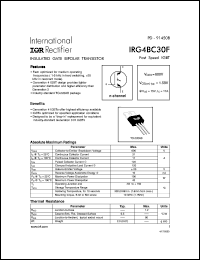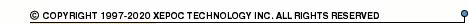More than479 017 registered clientsIRG4BC30 series datasheets. Manufacturer: International Rectifier.

 IRG4BC30 Insulated gate bipolar transistor. VCES = 600V, VCE(on)typ. = 1.59V @ VGE = 15V, IC = 17A in 3-pin TO-220AB package. Operational temperature range from -55°C to 150°C. Datasheet*) IRG4BC30FD Insulated gate bipolar transistor with ultrafast soft recovery diode. VCES = 600V, VCE(on)typ. = 1.59V @ VGE = 15V, IC = 17A in 3-pin TO-220AB package. Operational temperature range from -55°C to 150°C. Datasheet*) IRG4BC30K-S Insulated gate bipolar transistor. VCES = 600V, VCE(on)typ. = 2.21V @ VGE = 15V, IC = 16A in 3-pin DDPak package. Operational temperature range from -55°C to 150°C. Datasheet*) IRG4BC30K Insulated gate bipolar transistor. VCES = 600V, VCE(on)typ. = 2.21V @ VGE = 15V, IC = 16A in 3-pin TO-220AB package. Operational temperature range from -55°C to 150°C. Datasheet*) IRG4BC30KD Insulated gate bipolar transistor with ultrafast soft recovery diode. VCES = 600V, VCE(on)typ. = 2.21V @ VGE = 15V, IC = 16A in 3-pin TO-220AB package. Operational temperature range from -55°C to 150°C. Datasheet*) IRG4BC30KD-S Insulated gate bipolar transistor with ultrafast soft recovery diode. VCES = 600V, VCE(on)typ. = 2.21V @ VGE = 15V, IC = 16A in 3-pin DDPak package. Operational temperature range from -55°C to 150°C. Datasheet*) IRG4BC30S-S Insulated gate bipolar transistor. VCES = 600V, VCE(on)typ. = 1.4V @ VGE = 15V, IC = 18A in 3-pin DDPak package. Operational temperature range from -55°C to 150°C. Datasheet*) IRG4BC30S Insulated gate bipolar transistor. VCES = 600V, VCE(on)typ. = 1.4V @ VGE = 15V, IC = 18A in 3-pin TO-220AB package. Operational temperature range from -55°C to 150°C. Datasheet*) IRG4BC30U-S Insulated gate bipolar transistor. VCES = 600V, VCE(on)typ. = 1.95V @ VGE = 15V, IC = 12A in 3-pin DDPak package. Operational temperature range from -55°C to 150°C. Datasheet*) IRG4BC30U Insulated gate bipolar transistor. VCES = 600V, VCE(on)typ. = 1.95V @ VGE = 15V, IC = 12A in 3-pin TO-220AB package. Operational temperature range from -55°C to 150°C. Datasheet*) IRG4BC30UD Insulated gate bipolar transistor with ultrafast soft recovery diode. VCES = 600V, VCE(on)typ. = 1.95V @ VGE = 15V, IC = 12A in 3-pin TO-220AB package. Operational temperature range from -55°C to 150°C. Datasheet*) IRG4BC30W-S Insulated gate bipolar transistor. VCES = 600V, VCE(on)typ. = 2.10V @ VGE = 15V, IC = 12A in 3-pin DDPak package. Operational temperature range from -55°C to 150°C. Datasheet*) IRG4BC30W Insulated gate bipolar transistor. VCES = 600V, VCE(on)typ. = 2.70V @ VGE = 15V, IC = 12A in 3-pin TO-220AB package. Operational temperature range from -55°C to 150°C. Datasheet*) IRG4BC30F INSULATED GATE BIPOLAR TRANSISTOR(Vces=600V, Vce(on)typ.=1.59V, @Vge=15V, Ic=17A) Datasheet*) IRG4BC30KD-STRR INSULATED GATE BIPOLAR TRANSISTOR WITH ULTRAFAST SOFT RECOVERY DIODE(Vces=600V, Vce(on)typ.=2.21V, @Vge=15V, Ic=16A) Datasheet*) IRG4BC30KDS INSULATED GATE BIPOLAR TRANSISTOR WITH ULTRAFAST SOFT RECOVERY DIODE(Vces=600V, Vce(on)typ.=2.21V, @Vge=15V, Ic=16A) Datasheet*) IRG4BC30KS INSULATED GATE BIPOLAR TRANSISTOR(Vces=600V, Vce(on)typ.=2.21V, @Vge=15V, Ic=16A) Datasheet*) IRG4BC30SS INSULATED GATE BIPOLAR TRANSISTOR Standard Speed IGBT(Vces=600V, Vce(on)typ.=1.4V, @Vge=15V, Ic=18A) Datasheet*) IRG4BC30US INSULATED GATE BIPOLAR TRANSISTOR UltraFast Speed IGBT(Vces=600V, Vce(on)typ. = 1.95V, @Vge=15V, Ic=12A) Datasheet*) IRG4BC30WS INSULATED GATE BIPOLAR TRANSISTOR(Vces=600V, Vce(on)typ.=2.10V, @Vge=15V, Ic=12A) Datasheet*)